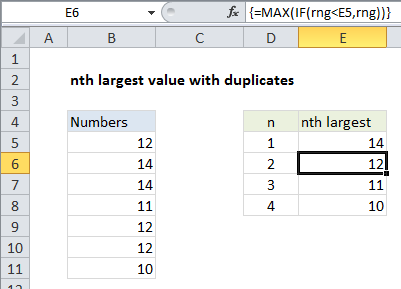## Excel Office

Excel How Tos, Tutorials, Tips & Tricks, Shortcuts

# nth largest value with duplicates in Excel

This tutorial shows how to calculate nth largest value with duplicates in Excel using the example below;

## Formula

```=MAX(IF(range<A2,range))
```## Explanation

To get the nth largest value in a data set with duplicates, you can use an array formula based on the MAX and IF functions.

Note: the LARGE function will easily return nth values, but LARGE will return duplicates when they exist in the source data.

Also See:   Calculate cap percentage at specific amount in Excel

In the example shown, the formula in E6 is:

`=MAX(IF(range<E5,range))`

Where  range is B5:B11

### How this formula works

First, we get the largest value using the MAX function in E5:

`=MAX(range)`

Once we have the largest value established, we create another formula that simply checks all values in the named range  against the “last largest value”:

`=MAX(IF(range<E5,range))`

Note: this is an array formula and must be entered with control + shift + enter.

Also See:   nth largest value in Excel

Any value that is lower than the “last largest” survives the test, and any value that’s not ends up FALSE. The resulting array looks like this:

`{12;FALSE;FALSE;11;12;12;10}`

As the formula is copied down the column, E5 increments at each row, so that MAX and IF return a new array. The MAX function continues to return the largest value in each array — the nth value in the series.

Also See:   Calculate total hours that fall between two times in Excel